# Automate Testing SSAS Tabular Models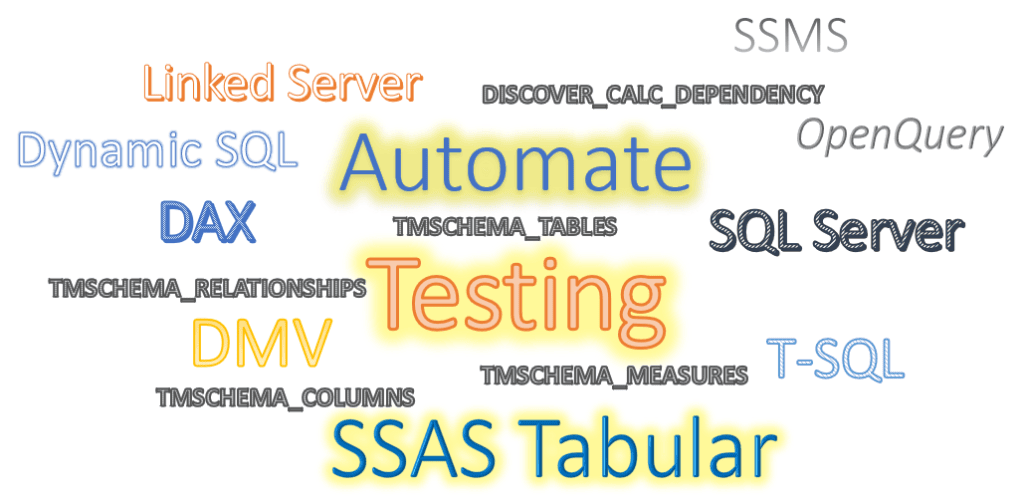In real world SSAS Tabular projects, you need to run many different testing scenarios to prove your customer that the data in Tabular model is correct. If you are running a Tabular Model on top of a proper data warehouse then your life would be a bit easier than when you build your semantic model on top of an operational database. However it would be still a fairly time-consuming process to run many test cases on Tabular Model, then run similar tests on the data warehouse and compare the results. So your test cases always have two sides, one side is your source database that can be a data warehouse and the other side is the Tabular Model. There are many ways to test the system, you can browse your Tabular Model in Excel, connecting to your Data Warehouse in Excel and create pivot tables then compare the data coming from Tabular Model and the data coming from the Data Warehouse. But, for how many measures and dimensions you can do the above test in Excel?

The other way is to run DAX queries on Tabular Model side. If your source database is a SQL Server database, then you need to run T-SQL queries on the database side then match the results of both sides to prove the data in Tabular Model is correct.

In this post I’d like to share with you a way to automate the DAX queries to be run on a Tabular model.

Straight away, this is going to be a long post, so you can make or take a cup of coffee while enjoying your reading.

While I will not cover the other side, the source or the data warehouse side, it is worth to automate that part too as you can save heaps of times. I’m sure a similar process can be developed in SQL Server side, but, I leave that part for now. What I’m going to explain in this post is just one of many possible ways to generate and run DAX queries and store the results in SQL Server. Perhaps it is not perfect, but, it is a good starting point. If you have a better idea it would be great to share it with us in the comments section below this post.

# Requirements

• SQL Server Analysis Services Tabular 2016 and later (Compatibility Level 1200 and higher)
• An instance of SQL Server
• SQL Server Management Studio (SSMS)

# How does it work

What I’m going to explain is very simple. I want to generate and run DAX queries and capture the results. The first step is to get all measures and their relevant dimensions, then I slice all the measures by all relevant dimensions and get the results. At the end I capture and store the results in a SQL Server temp table. Let’s think about a simple scenario:

• you have just one measure, [Internet Sales], from ‘Internet Sales’ table
• The measure is related to just one dimension, “Date” dimension
• The “Date” dimension has only four columns, Year, Month, Year-Month and Date
• you want to slice [Internet Sales] by Year, Month, Year-Month and Date

So you need to write four DAX queries as below:

```EVALUATE
SUMMARIZE(
'Internet Sales'
, Date'[Calendar Year]
, "Internet Sales", [Internet Total Sales]
)```
```EVALUATE
SUMMARIZE(
'Internet Sales'
, 'Date'[Month Name]
, "Internet Sales", [Internet Total Sales]
)```
```EVALUATE
SUMMARIZE(
'Internet Sales'
, 'Date'[Year-Month]
, "Internet Sales", [Internet Total Sales]
)```
```EVALUATE
SUMMARIZE(
'Internet Sales'
, 'Date'[Date]
, "Internet Sales", [Internet Total Sales]
)```

It is easy isn’t it? But, wait. What if you have 10 measures related to 4 dimension and each dimension has 10 columns? That sounds laborious doesn’t it? Well, in real world scenarios you won’t slice all measures by all relevant dimensions, but, you still need to do a lot. What we are going to do is to generate and run the DAX queries and store the results in a table in SQL Server. How cool is that?

OK, this is how it works…

• Creating a Linked Server for SSAS Tabular instance from SQL Server
• Generating DAX queries using Tabular DMVs
• Running the queries through Tabular model and getting/storing the results in a SQL Server temp table

## Creating Linked Server for SSAS Tabular (OLAP Service)

I’m not going to too much details on this. You can find a lot of resources over the internet on how to create a Linked Server for an instance of SSAS in SQL Server. Here  is the way you can create a Lined Server for SSAS from SSMS GUI:

• Open SSMS and connect to an instance of SQL Server
• Expand “Server Objects”
• Right click “Linked Servers”
• Click “New Linked Server…”• In the “New Linked Server” window, under “General” pane, enter a name for the linked server
• Make sure you select “Microsoft OLE DB Provider for Analysis Services”
• Enter the SSAS Server in the “Location” section
• Enter a desired database name in the “Catalog” section
• Click “Security” pane
• Click “Add” button and select a “Local Login” from the dropdown list
• Tick “Impersonate”
• Click “Be made using the login’s current security context” then click OKNote: Your security setting may be different from above.

# Generating DAX queries using Tabular DMVs

Now that we got our linked server sorted, let’s run some queries using the linked server and make sure it’s working as expected. The query structure for an SSAS Linked Server is as below:

select * from openquery([LINKED_SERVER_NAME], DESTINATION QUERY LANGUAGE)

As a simple test I run the following query which indeed is passing the DAX query to the Tabular model to run and retrieve the results:

```select *
from openquery([TABULAR2017], 'EVALUATE ''Date''')```

The above query brings all values from the ‘Date’ table from Tabular model into SQL Server. The results obviously can be stored in any sort of normal SQL tables.

Let’s have a closer look at the above query:

We have to use OPENQUERY to pass the DAX query through the Linked Server, run it in Tabular side and get the results. OPENQUERY  accepts DAX query in string format on the second argument. As we put table names in a single quote in DAX then we have to add an additional single quote to the table name as shown in the screenshot below.Before we continue let’s see what DAX query construction we need and what the query pattern we are after. This is what we get if we run all the DAX queries that mentioned earlier in this article, in a batch, yes! We can run multiple DAX queries in a single run when using Linked Server, which is not a surprise. From SSMS viewpoint we are just running a batch of SQL statements, aren’t we?

```select *
from openquery([TABULAR2017]
, 'EVALUATE SUMMARIZE(''Internet Sales''
, ''Date''[Calendar Year]
, "Internet Sales", [Internet Total Sales])')

select *
from openquery([TABULAR2017]
, 'EVALUATE SUMMARIZE(''Internet Sales''
, ''Date''[Month Name]
, "Internet Sales", [Internet Total Sales])')

select *
from openquery([TABULAR2017]
, 'EVALUATE SUMMARIZE(''Internet Sales''
, ''Date''[Year-Month]
, "Internet Sales", [Internet Total Sales])')

select *
from openquery([TABULAR2017]
, 'EVALUATE SUMMARIZE(''Internet Sales''
, ''Date''[Date]
, "Internet Sales", [Internet Total Sales])')```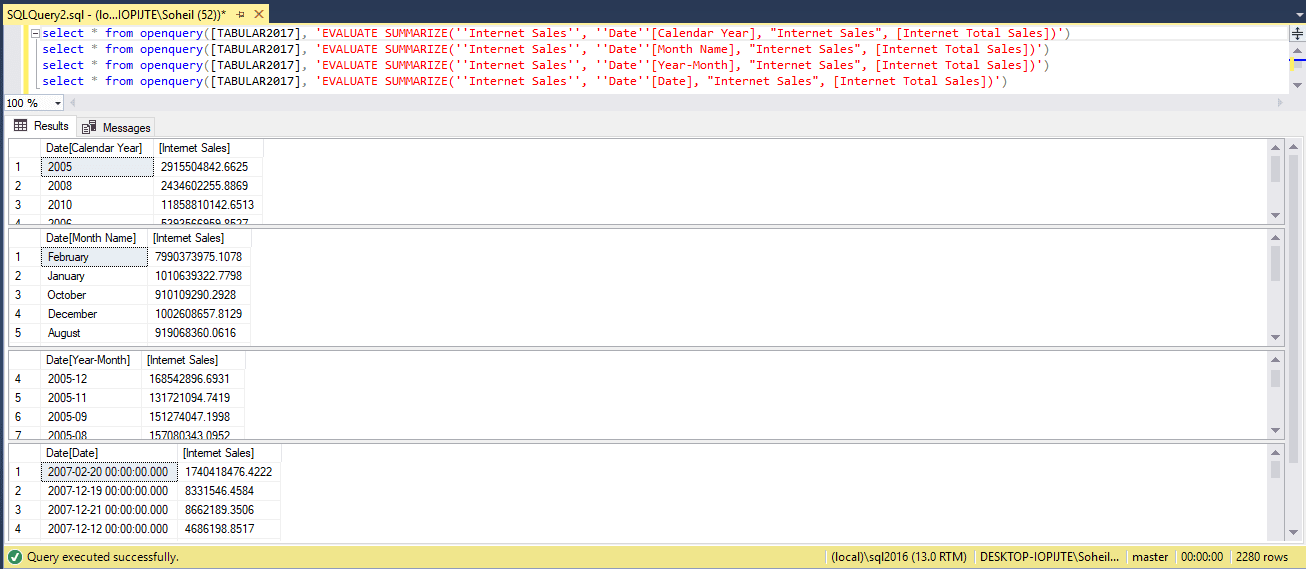This is a generic version of the above queries:

select * from openquery([TABULAR2017], ‘EVALUATE SUMMARIZE(”FACT_TABLE”, ”RELATED_DIMENSION”[COLUMN_NAME], MEASURE_GIVEN_NAME, [MEASURE_NAME])’)

As you see we have the following pattern repeated in all queries:

• A “SELECT” statement with “OPENQUERY” along with the linked server name
• In the query argument we have “EVALUATE SUMMARIZE(“

Then we have:

• two single quotes
• FACT_TABLE: the table that hosts the measure
• two single quotes and a comma
• another two single quotes
• RELATED_DIMENSION: this is a dimension tables which has a related to the measure
• again two single quotes
• open bracket
• COLUMN_NAME: the column from the dimension that is being used to slice the measure
• close bracket
• double quote, yes! this one is double qoute
• MEASURE_GIVEN_NAME: this is the name that we gave to the measure, like an alias
• double quote
• open bracket
• close bracket
• a close parentheses
• a last single quote
• and finally another close parentheses

So far we just ran a DAX query from SQL Server through a Linked Server, in the next few lines we will run DMVs to get the metadata we need to generate the DAX queries and run them from SQL Server through the Linked Server.  To generate the DAX query with the above pattern we need the following five DMVs:

• DISCOVER_CALC_DEPENDENCY
• TMSCHEMA_TABLES
• TMSCHEMA_MEASURES
• TMSCHEMA_COLUMNS
• TMSCHEMA_RELATIONSHIPS

Read more about Dynamic Management Views (DMVs) here.

While we don’t need all columns from the DMVs, I select just the columns we need and I also put some conditions in the where clause that I explain the reason for using those conditions later on. But for now the queries that we are after look like below DMV queries:

```select [Object]
, [Expression]
, [Referenced_Table]
from \$SYSTEM.DISCOVER_CALC_DEPENDENCY
where [Object_Type] = 'measure'```
```select [Name]
, [ID]
from \$SYSTEM.TMSCHEMA_TABLES
where not IsHidden```
```select [TableID]
, [Name]
, [Expression]
from \$SYSTEM.TMSCHEMA_MEASURES
where not IsHidden
and [DataType] <> 2```
```select [TableID]
, [ExplicitName]
from \$SYSTEM.TMSCHEMA_COLUMNS
where not [IsHidden]
and [Type] <> 3
and not [IsDefaultImage]
and [ExplicitDataType] = 2
and [State] = 1```
```select [FromTableID]
, [ToTableID]
from \$SYSTEM.TMSCHEMA_RELATIONSHIPS
where IsActive```

As you see I used some enumerations in the above queries as below:

• In TMSCHEMA_MEASURES, “DataType” shows the data type of the measure. The possible values are:
 Enumeration Description 2 String 6 Int64 8 Double 9 DateTime 10 Decimal 11 Boolean 17 Binary 19 Unknown (the measure is in an Error state) 20 Variant (measure with varying data type)

So adding “DataType <> 2” to the where clause when querying TMSCHEMA_MEASURES means that we are NOT interested in textual measures like when you define a measure to show the user name using USERNAME() function in DAX.

• In TMSCHEMA_COLUMNS, I used “Type”, “ExplicitDataType” and “State” enumerations. The possible values for the above enumerations are:
 Name Enumeration Description Type 1 Data (Comes from data source) 2 Calculated (Calculated Column) 3 RowNumber (This is an internal column that is NOT visible. It represents the row number.) 4 CalculatedTableColumn (A calculated column in a calculated table) ExplicitDataType 1 Automatic (When calculated columns or calculated table columns set the value to Automatic, the type is automatically inferred) 2 String 6 Int64 8 Double 9 DateTime 10 Decimal 11 Boolean 17 Binary 19 Unknown (The column is in an Error state) State 1 Ready (The column is queryable and has up-to-date data) 3 NoData (The column is still queryable) 4 CalculationNeeded (The column is not queryable and needs to be refreshed) 5 SemanticError (The column is in an Error state because of an invalid expression) 6 EvaluationError (The column is in an Error state because of an error during expression evaluation) 7 DependencyError (The column is in an error state because some of its calculation dependencies are in an error state) 8 Incomplete (Some parts of the column have no data, and the column needs to be refreshed to bring the data in) 9 SyntaxError (The column is in an error state because of a syntax error in its expression)

So adding “Type <> 3  and ExplicitDataType = 2  and State = 1” to the where clause when querying “TMSCHEMA_COLUMNS” means that we are only interested in the columns that are NOT internal row numbers and their data type is string and they are queryable and ready to use.

The next step is to put the above queries in the OPENQUERY. The queries at the end will look like the below queries:

```select [Object] MeasureName
, [Expression]
, [Referenced_Table] ReferencedTable
from openquery([TABULAR2017]
, 'select [Object]
, [Expression]
, [Referenced_Table]
from \$SYSTEM.DISCOVER_CALC_DEPENDENCY
where [Object_Type] = ''measure'''
)```
```select [TableID]
, [Name] MeasureName
, [Expression]
from openquery([TABULAR2017]
,  'select [TableID]
, [Name]
, [Expression]
from \$SYSTEM.TMSCHEMA_MEASURES
where not [IsHidden]
and [DataType] <> 2'
)```
```select [FromTableID]
, [ToTableID]
from openquery([TABULAR2017], 'select [FromTableID]
, [ToTableID]
from \$SYSTEM.TMSCHEMA_RELATIONSHIPS
where [IsActive]'
)```
```select [Name] TableName
, [ID]
from openquery([TABULAR2017], 'select [Name]
, [ID]
from \$SYSTEM.TMSCHEMA_TABLES
where not IsHidden'
)```
```select [TableID]
,  [ExplicitName] RelatedColumn
from openquery([TABULAR2017], 'select [TableID]
, [ExplicitName]
from \$SYSTEM.TMSCHEMA_COLUMNS
where not [IsHidden]
and [Type] <> 3
and not [IsDefaultImage]
and [ExplicitDataType] = 2
and [State] = 1'
)```

Now we want to join the above tables to get:

• Visible measures
• The base tables used in measures (referenced tables)
• Related dimension to the measures
• Columns of those related dimensions

Having the four above elements we can dynamically generate the DAX query that we want by joining the above five queries. I used CTE construction to join the above queries:

```;with
MeasureReferences as (
select [Object] MeasureName
, [Expression]
, [Referenced_Table] ReferencedTable
from openquery([TABULAR2017], 'select [Object]
, [Expression]
, [Referenced_Table]
from \$SYSTEM.DISCOVER_CALC_DEPENDENCY
where [Object_Type] = ''measure'' '
)
)
, Measures as (
select [TableID]
, [Name] MeasureName
, [Expression]
from openquery([TABULAR2017], 'select [TableID]
, [Name]
, [Expression]
from \$SYSTEM.TMSCHEMA_MEASURES
where not [IsHidden] and [DataType] <> 2'
)
where  charindex('SUM', ltrim(rtrim(cast([Expression] as varchar(max))))) = 1
)
, Relationships as (
select [FromTableID]
, [ToTableID]
from openquery([TABULAR2017], 'select [FromTableID]
, [ToTableID]
from \$SYSTEM.TMSCHEMA_RELATIONSHIPS
where [IsActive]'
)
)
, Tables as (
select [Name] TableName
, [ID]
from openquery([TABULAR2017], 'select [Name]
, [ID]
from \$SYSTEM.TMSCHEMA_TABLES
where not IsHidden'
)
)
, Columns as (
select [TableID]
,  [ExplicitName] RelatedColumn
from openquery([TABULAR2017], 'select [TableID]
, [ExplicitName]
from \$SYSTEM.TMSCHEMA_COLUMNS
where not [IsHidden]
and [Type] <> 3
and not [IsDefaultImage]
and [ExplicitDataType] = 2
and [State] = 1'
)
)

select cast(mr.ReferencedTable as varchar(max)) TableName
, cast(m.MeasureName as varchar(max)) MeasureName
, cast((select TableName
from Tables
where [ID] = r.[ToTableID]
) as varchar(max)
) RelatedDimension
, cast(c.RelatedColumn as varchar(max)) RelatedColumn
from Measures m
join MeasureReferences mr on cast(mr.MeasureName as varchar(max)) = cast(m.MeasureName as varchar(max))
join Relationships r on (select ID
from Tables
where cast(mr.ReferencedTable as varchar(max)) = cast(TableName as varchar(max))
) = r.[FromTableID]
join Columns c on c.[TableID] = r.[ToTableID]```Let’s revisit the DAX query that we are going to generate using the results of the above query.

```EVALUATE
SUMMARIZE(
'Internet Sales'
, 'Date'[Calendar Year]
, "Internet Sales", [Internet Total Sales]
)```

If we run the above query this is what we get: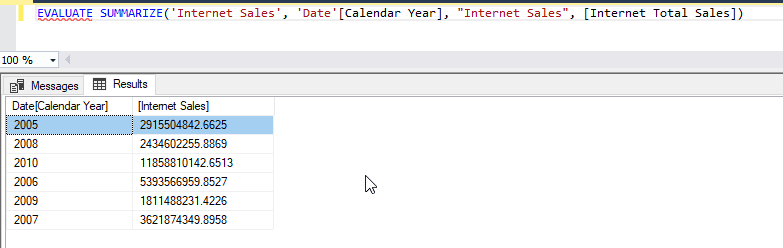That looks fine, but, when we generate DAX queries, we will automatically detect all related dimensions to all measures and generate the query so that it slices each measure by every single columns of related dimensions. In that case our column names will be different from what we see in the above screenshot for each query that we run.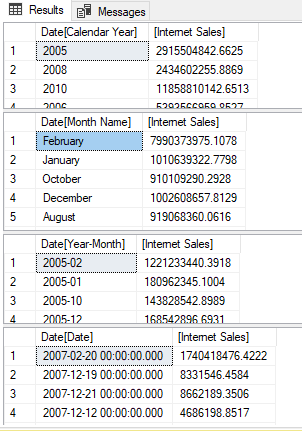So we have to hard-code the column names which is not ideal. In addition, we are going to insert that data in a SQL Server table. With hardcoded column names then we will have some meaningless dimension values in the left column and some measure values in the right column. Therefore, we have to change the above query a little bit so that it dynamically use the column names as values for two additional columns. So the result of the query brings 4 columns, the first column (from the left) contains the column name along with its value next to it in the second column. The third column shows the measure name and the fourth column shows the measure values.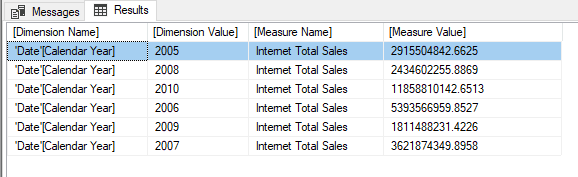This looks much better. I ran the following DAX query to get the above result:

```EVALUATE
SELECTCOLUMNS (
SUMMARIZE (
'Internet Sales'
, 'Date'[Calendar Year]
, "Measure Name", "Internet Sales"
, "Value", [Internet Total Sales]
),
"Dimension Name", "'Date'[Calendar Year]"
, "Dimension Value", 'Date'[Calendar Year]
, "Measure Name", "Internet Total Sales"
, "Measure Value", [Internet Total Sales]
)```

The next step is to dynamically generate the latter DAX query using the results of DMVs running through the Linked Server.

In the following query we define a local variable to generate the DAX query, then we use “Print” T-SQL function to see the results.

Note: The “Print” function has a limitation on displaying large strings, so we will only a portion of the results. Read more about “Print” here.

```declare @SQL varchar(max) = null

;with
MeasureReferences as (
select [Object] MeasureName
, [Expression]
, [Referenced_Table] ReferencedTable
from openquery([TABULAR2017], 'select [Object]
, [Expression]
, [Referenced_Table]
from \$SYSTEM.DISCOVER_CALC_DEPENDENCY
where [Object_Type] = ''measure'' '
)
)
, Measures as (
select [TableID]
, [Name] MeasureName
, [Expression]
from openquery([TABULAR2017], 'select [TableID]
, [Name]
, [Expression]
from \$SYSTEM.TMSCHEMA_MEASURES
where not [IsHidden] and [DataType] <> 2'
)
where  charindex('SUM', ltrim(rtrim(cast([Expression] as varchar(max))))) = 1
)
, Relationships as (
select [FromTableID]
, [ToTableID]
from openquery([TABULAR2017], 'select [FromTableID]
, [ToTableID]
from \$SYSTEM.TMSCHEMA_RELATIONSHIPS
where [IsActive]'
)
)
, Tables as (
select [Name] TableName
, [ID]
from openquery([TABULAR2017], 'select [Name]
, [ID]
from \$SYSTEM.TMSCHEMA_TABLES
where not IsHidden'
)
)
, Columns as (
select [TableID]
,  [ExplicitName] RelatedColumn
from openquery([TABULAR2017], 'select [TableID]
, [ExplicitName]
from \$SYSTEM.TMSCHEMA_COLUMNS
where not [IsHidden]
and [Type] <> 3
and not [IsDefaultImage]
and [ExplicitDataType] = 2
and [State] = 1'
)
)

select @SQL = ISNULL(@SQL, '') + 'select * from openquery ([TABULAR2017], ''EVALUATE SELECTCOLUMNS(SUMMARIZE ('''''+[TableName]+''''', '''''+RelatedDimension+'''''['+RelatedColumn+'], "Measure Name", "'+MeasureName+'", "Value", ['+MeasureName+']) , "Dimension Name", "'''''+RelatedDimension+'''''['+RelatedColumn+']", "Dimension Value", '''''+RelatedDimension+'''''['+RelatedColumn+'], "Measure Name",  "'+MeasureName+'", "Measure Value",  ['+MeasureName+'])'')
'
from (
select cast(mr.ReferencedTable as varchar(max)) TableName
, cast(m.MeasureName as varchar(max)) MeasureName
, cast((select TableName
from Tables
where [ID] = r.[ToTableID]
) as varchar(max)
) RelatedDimension
, cast(c.RelatedColumn as varchar(max)) RelatedColumn
from Measures m
join MeasureReferences mr on cast(mr.MeasureName as varchar(max)) = cast(m.MeasureName as varchar(max))
join Relationships r on (select ID
from Tables
where cast(mr.ReferencedTable as varchar(max)) = cast(TableName as varchar(max))
) = r.[FromTableID]
join Columns c on c.[TableID] = r.[ToTableID]
) as tbl

Print @SQL```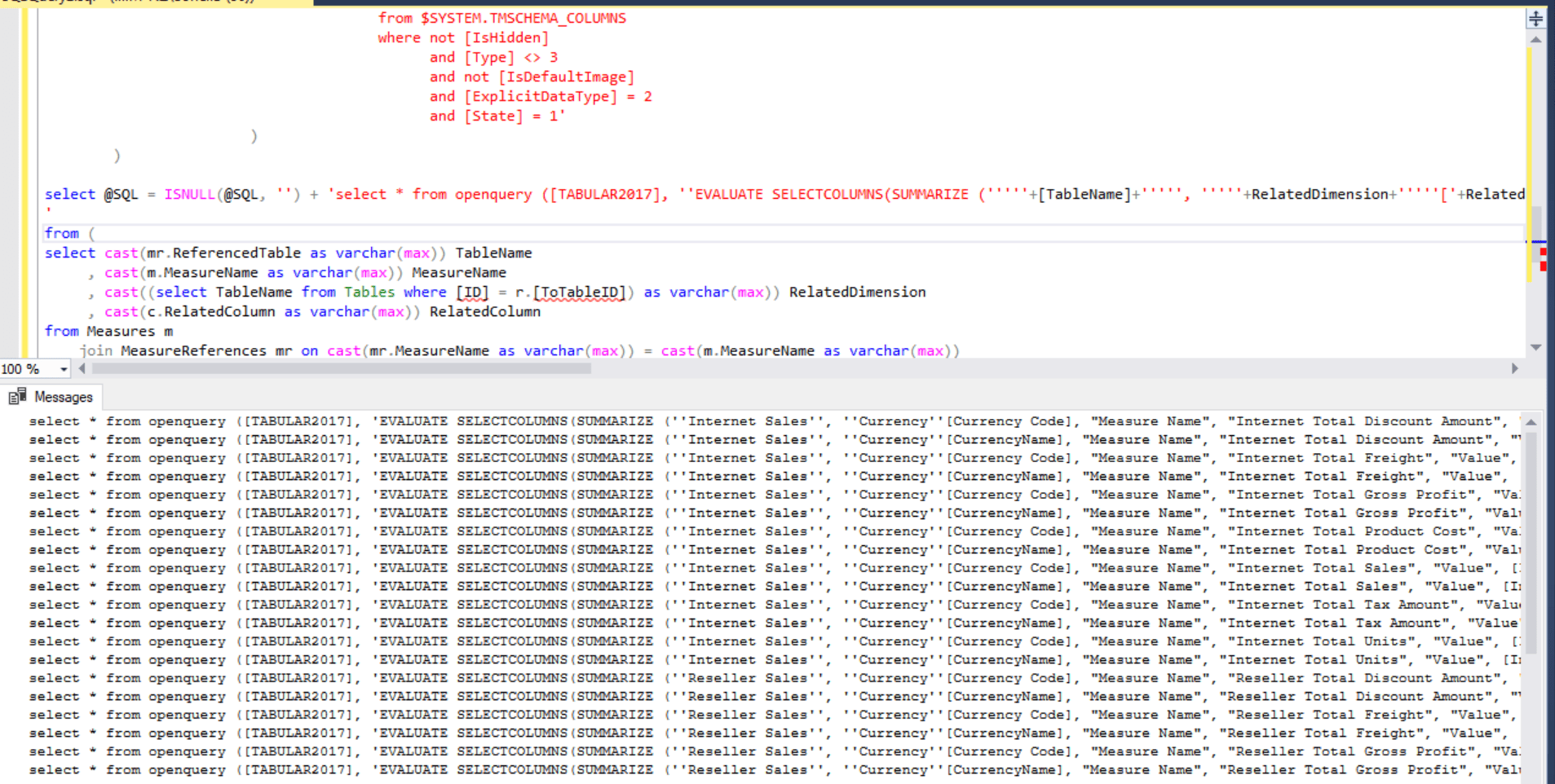You can copy/paste and run every query that is generated to get the results.

I manually ran the following query that is copied from the results:

`select * from openquery ([TABULAR2017], 'EVALUATE SELECTCOLUMNS(SUMMARIZE (''Internet Sales'', ''Currency''[Currency Code], "Measure Name", "Internet Total Sales", "Value", [Internet Total Sales]) , "Dimension Name", "''Currency''[Currency Code]", "Dimension Value", ''Currency''[Currency Code], "Measure Name", "Internet Total Sales", "Measure Value", [Internet Total Sales])')`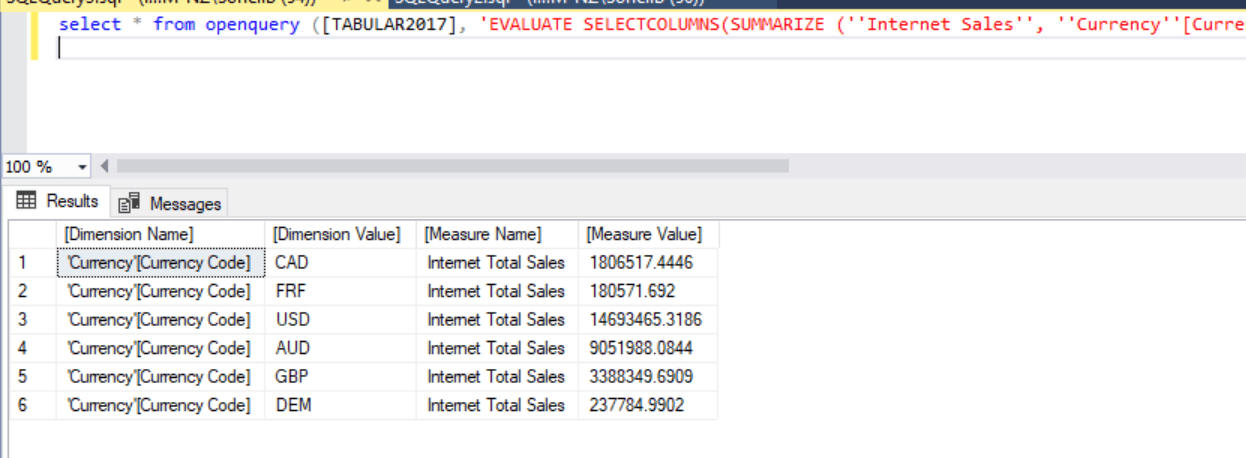The last step is to execute all generated queries and store the results in a SQL Server Table.

# Running Generated DAX Queries through Linked Server and Store the Results in SQL Server

This is an easy one. We just need to execute the dynamic SQL stored in @SQL local variable then we store the results in a table we create in SQL Server. For the sake of this post I create a global temporary table in SQL Server. So the final query will look like this:

```if object_id('tempdb..##Results') is not null drop table ##Results
create table ##Results (DimensionName varchar(max)
, DimensionValue varchar(max)
, MeasureName varchar(max)
, MeasureValue bigint
) --Create a global temp table
declare @SQL varchar(max) = null

;with --Get measures, their related dimensions and dimenion columns
MeasureReferences as (
select [Object] MeasureName
, [Expression]
, [Referenced_Table] ReferencedTable
from openquery([TABULAR2017], 'select [Object]
, [Expression]
, [Referenced_Table]
from \$SYSTEM.DISCOVER_CALC_DEPENDENCY
where [Object_Type] = ''measure'' '
)
)
, Measures as (
select [TableID]
, [Name] MeasureName
, [Expression]
from openquery([TABULAR2017], 'select [TableID]
, [Name]
, [Expression]
from \$SYSTEM.TMSCHEMA_MEASURES
where not [IsHidden] and [DataType] <> 2'
)
where  charindex('SUM', ltrim(rtrim(cast([Expression] as varchar(max))))) = 1
)
, Relationships as (
select [FromTableID]
, [ToTableID]
from openquery([TABULAR2017], 'select [FromTableID]
, [ToTableID]
from \$SYSTEM.TMSCHEMA_RELATIONSHIPS
where [IsActive]'
)
)
, Tables as (
select [Name] TableName
, [ID]
from openquery([TABULAR2017], 'select [Name]
, [ID]
from \$SYSTEM.TMSCHEMA_TABLES
where not IsHidden'
)
)
, Columns as (
select [TableID]
,  [ExplicitName] RelatedColumn
from openquery([TABULAR2017], 'select [TableID]
, [ExplicitName]
from \$SYSTEM.TMSCHEMA_COLUMNS
where not [IsHidden]
and [Type] <> 3
and not [IsDefaultImage]
and [ExplicitDataType] = 2
and [State] = 1'
)
)

select @SQL = ISNULL(@SQL, '') + 'select * from openquery ([TABULAR2017], ''EVALUATE SELECTCOLUMNS(SUMMARIZE ('''''+[TableName]+''''', '''''+RelatedDimension+'''''['+RelatedColumn+'], "Measure Name", "'+MeasureName+'", "Value", ['+MeasureName+']) , "Dimension Name", "'''''+RelatedDimension+'''''['+RelatedColumn+']", "Dimension Value", '''''+RelatedDimension+'''''['+RelatedColumn+'], "Measure Name",  "'+MeasureName+'", "Measure Value",  ['+MeasureName+'])'')
'
from (
select cast(mr.ReferencedTable as varchar(max)) TableName
, cast(m.MeasureName as varchar(max)) MeasureName
, cast((select TableName
from Tables
where [ID] = r.[ToTableID]
) as varchar(max)
) RelatedDimension
, cast(c.RelatedColumn as varchar(max)) RelatedColumn
from Measures m
join MeasureReferences mr on cast(mr.MeasureName as varchar(max)) = cast(m.MeasureName as varchar(max))
join Relationships r on (select ID
from Tables
where cast(mr.ReferencedTable as varchar(max)) = cast(TableName as varchar(max))
) = r.[FromTableID]
join Columns c on c.[TableID] = r.[ToTableID]
) as tbl --Generate DAX queries dynamically
insert into ##Results
execute (@SQL) --Execute the DAX queries

select DimensionName, DimensionValue, MeasureName, FORMAT(MeasureValue, '#,#.#') MeasureValue
from ##Results
where MeasureValue <> 0 and MeasureValue is not null
```

# Considerations

The above query should work on any Tabular Model if you setup the linked server correctly. However, as you may noticed, it will generate a lot of queries for all possible combinations of slicing a measure with all columns from all related dimensions. The queries will run against an instance of SSAS Tabular one by one. Therefore, if you have a lot of measures and dimensions, then for sure you’ll face performance issues. Unfortunately this is the case in real world projects. But, what you can do is to pick some of the most dimensions that are the most important ones to the business and restrict the above query to generate only some possibilities. The other point is that in lots of cases you really don’t need to test all combinations of measures and all columns from related dimensions. So you can add some more conditions to the query to generate less queries as desired. For instance, in the above query, look at the “Measures” CTE. I put a condition in to get only the measures that their expressions start with “SUM”. The reason for that is that I wanted to get only the measures that are basically summation base measures. In real world projects you may have hundreds of measures and running the above query without any conditions doesn’t sound quite right.

# Possible Questions

Q: Is the query specific to SSAS Tabular Model?

A: Yes, it is. But, you can do something similar for SSAS Multidimensional.

Q: Is this method dependent on the Tabular server name and/or database name?

A: As far as you setup the Linked Server correctly there must not be any issues.

Q: Can we use this method for testing Power BI models?

A: Yes. You just need to open your Power BI Desktop (pbix) file and find its local port number. Then you can create a Linked Server to your Power BI file and then you’re good to go. Read more about finding Power BI Desktop Local Port number here.

Q: This method is only to get measures and their related dimensions’ columns in SSAS Tabular side. At last we have to compare the results with the underlying data source(s) like a data warehouse. How should we test against the source systems.

A: As mentioned earlier, we are only covering the SSAS Tabular side. You can do something similar in your data warehouse side and compare the results. One of the challenges would be finding the column mappings between your data warehouse and the SSAS Tabular model. There is a “SourceColumn” available in the “\$SYSTEM.TMSCHEMA_COLUMNS” DMV to get the source column names. That can be a good starting point. Then you can use dynamic SQL to generate the queries and run against your data warehouse, getting the results in a SQL Server table. The rest would be easy to compare the two results.

Q: Is this method valid for Azure Analysis Services too?

A: Yes it is.

This site uses Akismet to reduce spam. Learn how your comment data is processed.